Refer to our Texas Go Math Grade 1 Answer Key Pdf to score good marks in the exams. Test yourself by practicing the problems from Texas Go Math Grade 1 Lesson 6.3 Answer Key Use Doubles to Add.

Essential Question
Explanation:
To get a double of a number,
we add the same number to itself.
For example, double of 2 is 2 + 2 = 4.

Explore
Draw to show the problem.
Write the number of fish.Lesson Check

For The Teacher

• Read the following problem. There are 3 orange fish, 3 striped fish, and 1 white fish in the class fish tank. How many fish are in the fish tank?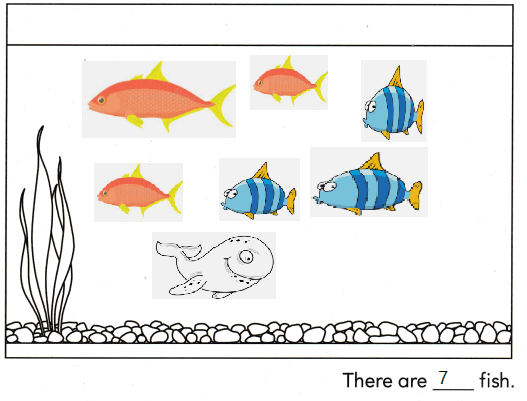Explanation:
There are 3 orange fish, 3 striped fish,
3 + 3 = 1
and 1 white fish in the class fish tank.
6 + 1 = 7
7 fish are in the fish tank
Math Talk

Mathematical Processes
How does knowing 3 + 3 help you solve the problem? Explain.
Explanation:
3 + 3 is known as a double fact
A number is added to itself is called a double fact

Model and DrawExplanation: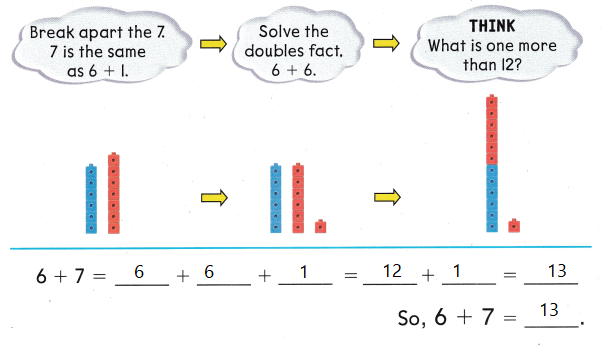Explanation:
6 + 7
is made as double plus one
6 + 6 + 1 = 13

Share and Show

Useto model. Make doubles. Add.

Question 1.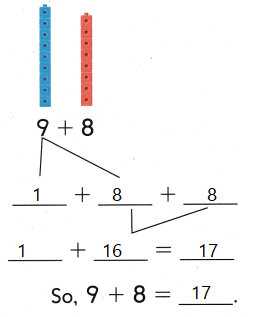Explanation:
9 + 8
is made as double plus one
8 + 8 + 1 = 9

Question 2.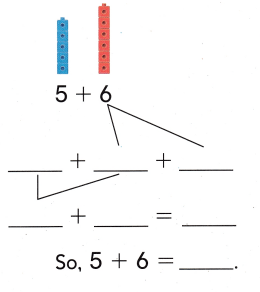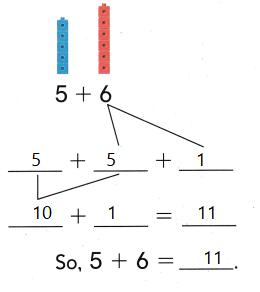Explanation:
5 + 6
is made as double plus one
5 + 5 + 1 = 11

Problem Solving
Use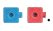Make doubles. Add.

Question 3.Explanation:
7 + 8
is made as double plus one
7 + 7 + 1 = 15

Question 4.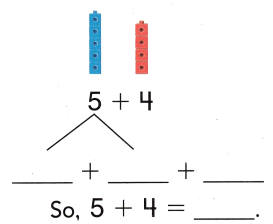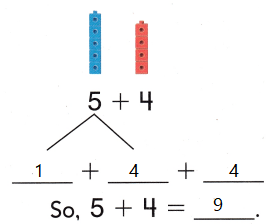Explanation:
5 + 4
is made as double plus one
4 + 4 + 1 = 9

Question 5.
Multi-Step Mandy has the same number of red and yellow leaves. Then she finds 1 more yellow leaf. There are 17 leaves in all. How many leaves are red? How many leaves are yellow?
_____ red leaves _____ yellow leaves
8 red leaves 9 yellow leaves
Explanation:
Total leaves are 17
8 +8 + 1 = 17
Double plus one
so, red leaves are 8 and yellow leaves are 8
8 + 1 = 9

H.O.T. Multi-Step Would you use count on or doubles to solve? Explain.

Question 6.
3 + 4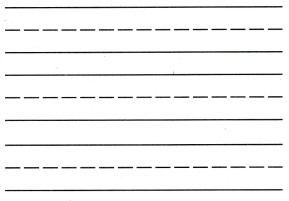Explanation:
3 + 4 is derived as double plus one
3 + 3 + 1  7

Question 7.
3 + 9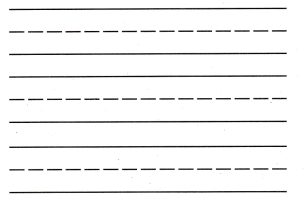Explanation:
3 + 9 = 12
started from greater number 9 and counted on

Question 8.
Leo walks in the park. He sees 3 dogs. He sees 3 cats. He sees 1 squirrel. How many animals does Leo see?(A) 3
(B) 7
(C) 6
Explanation:
Leo walks in the park.
He sees 3 dogs. He sees 3 cats.
3 + 3 = 6
He sees 1 squirrel.
6 + 1 = 7
7 animals that Leo see

Question 9.
Analyze There are 7 birds in a tree. 8 more birds fly over. Which has the same sum as 7 + 8?(A) 7 + 7 + 1
(B) 8 + 8 + 1
(C) 7 + 7 + 2
Explanation:
There are 7 birds in a tree. 8 more birds fly over.
7 + 7 + 1 = 15
Which has the same sum as 7 + 8
7 + 8 = 15

Question 10.
Multi-Step There are 5 white houses. There are 5 yellow houses. There is 1 brown house. How many houses
are there?
(A) 10
(B) 15
(C) 11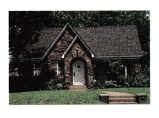Explanation:
There are 5 white houses. There are 5 yellow houses.
5 + 5 = 10
There is 1 brown house.
10 + 1 = 11
11 houses are there

Question 11.
Texas Test Prep Which has the same sum as 9 + 8?
(A) 6 + 6 + 2
(B) 1 + 8 + 8
(C) 2 + 8 + 8
Explanation:
1 + 8 + 8 =17
9 + 9 = 17
Both the sums are same.

Take Home Activity

• Ask your child to show you how to use what he or she knows about doubles to help solve 6 + 5.

### Texas Go Math Grade 1 Lesson 6.3 Homework and Practice Answer Key

Question 1.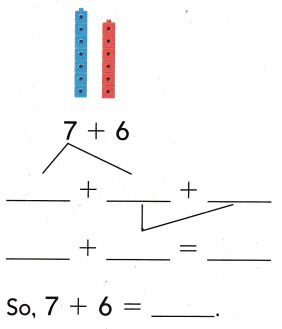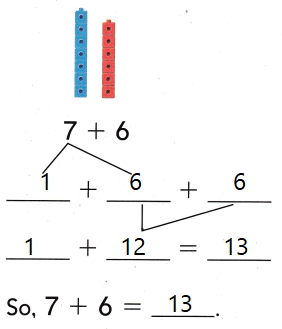Explanation:
6 + 7
is made as double plus one
6 + 6 + 1 = 13

Question 2.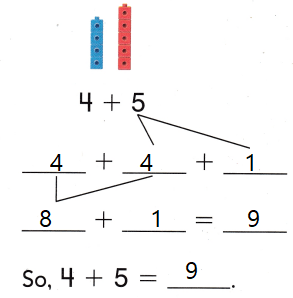Explanation:
4 + 5
is made as double plus one
4 + 4 + 1 = 5

Problem Solving

Make doubles. Show how you find the sum.

Question 3.
There are 4 ants on the step. 5 more ants join them. How many ants are on the step?
___ + ____ + ____ = ____ ants
4 + 4 + 1 = 9
Explanation:
4 + 5 is changed as double plus one.

Texas Test Prep

Lesson Check

Question 4.
Toby finds 6 red pencils and 6 blue pencils. Then he finds 1 yellow pencil How many pencils does Toby find?
(A) 12
(B) 13
(C) 7
Explanation:
Toby finds 6 red pencils and 6 blue pencils.
6 + 6 = 12
Then he finds 1 yellow pencil
12 + 1 = 13
13  pencils that Toby find

Question 5.
There are 8 fish in a pond. 9 more fish swim by. Which has the same sum as 8 + 9?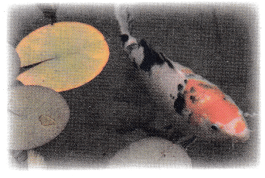(A) 8 + 8 + 1
(B) 9 + 9 + 1
(C) 8 + 8 + 2
Explanation:
There are 8 fish in a pond. 9 more fish swim by.
Which has the same sum as 8 + 9
is 8 + 8 + 1 = 17

Question 6.
Multi-Step George has the same number of black shirts as white shirts. Then he gets 1 more white shirt. He has 9 shirts in all. How many shirts are white?
(A) 10
(B) 4
(C) 5
Explanation:
Total shirts are 9
9 is changed as Double plus 1
4 + 4 + 1
White and black shirts are equal
so, 4 each
1 white shirt more
4 + 1 = 5

Question 7.
Which has the same sum as 5 + 6?
(A) 5 + 5 + 1
(B) 6 + 6 + 0
(C) 5 + 6 + 1